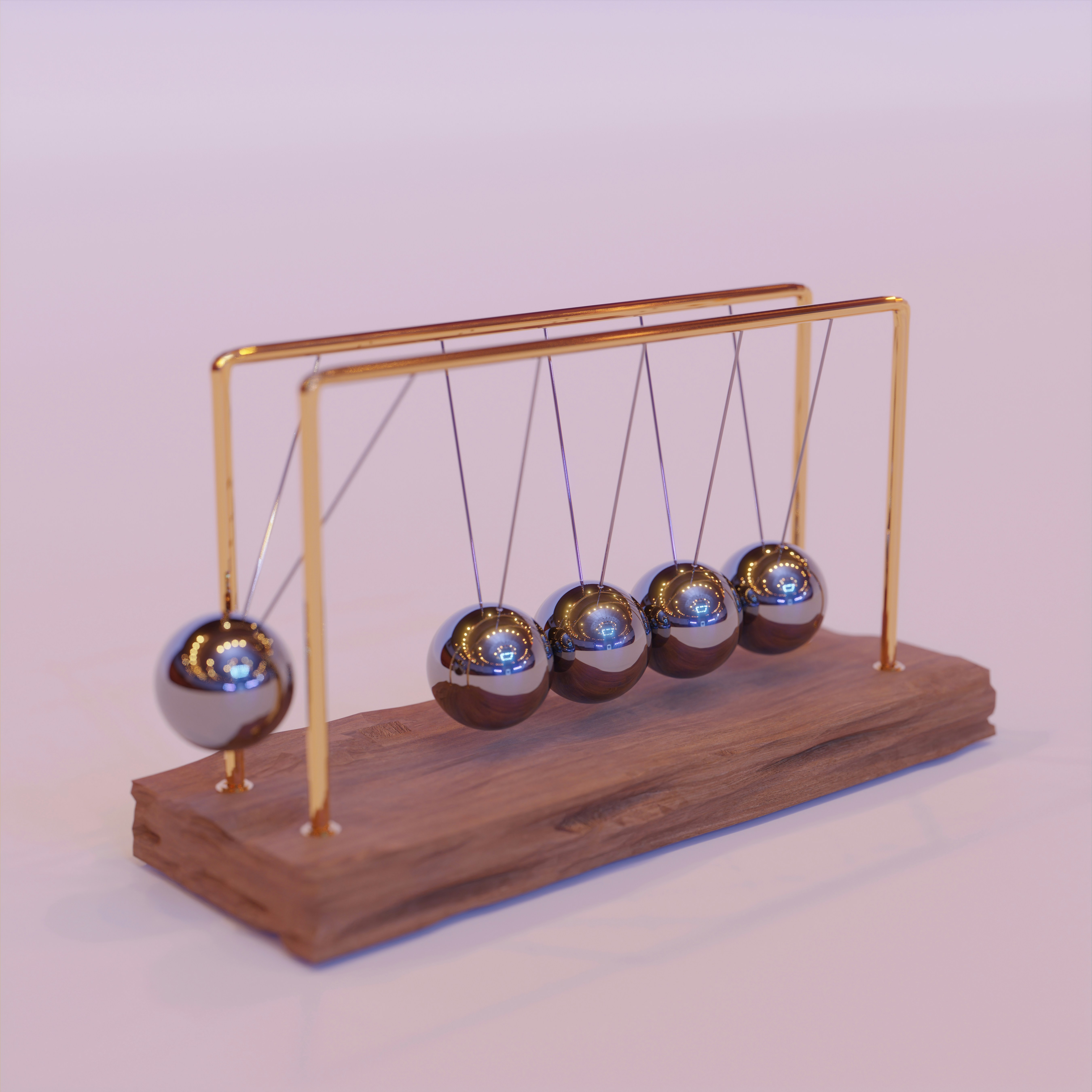# The Fascinating World of Classical Mechanics: An Exploration of Momentum

## Exploring the Fundamentals of Classical Mechanics: The Role of Momentum in Design and Prediction

By Sallabh KumarPublished 3 months ago 3 min read
LikePhoto by Sunder Muthukumaran on Unsplash

Classical mechanics is the study of the motion of objects under the influence of forces. It is a fundamental area of physics that has applications in many fields, from engineering to astronomy. One of the most important concepts in classical mechanics is momentum, which is a measure of an object's motion. In this article, we will explore the fascinating world of momentum and its applications in classical mechanics.

What is Momentum?

Momentum is defined as the product of an object's mass and its velocity. Mathematically, it can be represented as:

p = mv

Where p is the momentum, m is the mass of the object, and v is its velocity. Momentum is a vector quantity, meaning it has both magnitude and direction. In other words, an object's momentum is not just determined by how fast it is moving, but also by the direction it is moving in.

Applications of Momentum

Momentum is a fundamental concept in classical mechanics and has many practical applications. For example, it is used in the design of airbags in cars. Airbags work by rapidly inflating and deflating to cushion the impact of a collision. To do this effectively, the airbag needs to exert a force on the passenger that is proportional to the change in momentum of the passenger as they collide with the airbag.

Momentum is also used in sports, such as baseball and football. In baseball, pitchers use their body weight and the velocity of their throwing arm to increase the momentum of the ball they are throwing. In football, momentum is used to calculate the force of impact between players during a tackle.

Conservation of Momentum

One of the most important principles in classical mechanics is the conservation of momentum. This principle states that the total momentum of a system of objects remains constant, as long as no external forces act on the system. In other words, if two objects collide, the total momentum of the objects before the collision is equal to the total momentum after the collision.

The conservation of momentum is a powerful tool in classical mechanics and has many applications. For example, it can be used to predict the motion of planets and other celestial objects. By calculating the momentum of an object and its gravitational interactions with other objects, scientists can predict the future motion of the object with remarkable accuracy.

Another example of the conservation of momentum is the principle of action and reaction. This principle states that for every action, there is an equal and opposite reaction. This means that when two objects interact, the forces they exert on each other are equal and opposite. For example, when a rocket launches into space, it exerts a force on the gases that are expelled from its engines. The gases, in turn, exert an equal and opposite force on the rocket, propelling it forward.

Conclusion

Momentum is a fundamental concept in classical mechanics that has many practical applications in our daily lives. From designing airbags to predicting the motion of celestial objects, the study of momentum has contributed to many advancements in technology and our understanding of the world around us.

By understanding the principles of momentum and the conservation of momentum, we can gain a deeper appreciation for the laws of classical mechanics and the role they play in shaping our world. As we continue to push the boundaries of our understanding, the study of classical mechanics and the principles of momentum will undoubtedly play an important role in our ongoing quest for knowledge and progress.

science
Like

### Sallabh Kumar

How does it work?

There are no comments for this story

Be the first to respond and start the conversation.